# 5.4: Python Assignment

$$\newcommand{\vecs}{\overset { \rightharpoonup} {\mathbf{#1}} }$$ $$\newcommand{\vecd}{\overset{-\!-\!\rightharpoonup}{\vphantom{a}\smash {#1}}}$$$$\newcommand{\id}{\mathrm{id}}$$ $$\newcommand{\Span}{\mathrm{span}}$$ $$\newcommand{\kernel}{\mathrm{null}\,}$$ $$\newcommand{\range}{\mathrm{range}\,}$$ $$\newcommand{\RealPart}{\mathrm{Re}}$$ $$\newcommand{\ImaginaryPart}{\mathrm{Im}}$$ $$\newcommand{\Argument}{\mathrm{Arg}}$$ $$\newcommand{\norm}{\| #1 \|}$$ $$\newcommand{\inner}{\langle #1, #2 \rangle}$$ $$\newcommand{\Span}{\mathrm{span}}$$ $$\newcommand{\id}{\mathrm{id}}$$ $$\newcommand{\Span}{\mathrm{span}}$$ $$\newcommand{\kernel}{\mathrm{null}\,}$$ $$\newcommand{\range}{\mathrm{range}\,}$$ $$\newcommand{\RealPart}{\mathrm{Re}}$$ $$\newcommand{\ImaginaryPart}{\mathrm{Im}}$$ $$\newcommand{\Argument}{\mathrm{Arg}}$$ $$\newcommand{\norm}{\| #1 \|}$$ $$\newcommand{\inner}{\langle #1, #2 \rangle}$$ $$\newcommand{\Span}{\mathrm{span}}$$

Quantitative Structure-Property Relationships

QSPR.ipynb

Excel_multiple_linear_regression_assignment.docx

BP.csv

102BP.csv

• You can use the notebook you created in section 1.5 or  the Jupyter hub at LibreText: https://jupyter.libretexts.org (see your instructor if you do not have access to the hub).
• This page is an html version of the above .ipynb file.
• If you have questions on this assignment you should use this web page and the hypothes.is annotation to post a question (or comment) to the 2019OLCCStu class group.   Contact your instructor if you do not know how to access the 2019OLCCStu group within the hypothes.is system.
• You will need to have the BP.csv and 102BP.csv files in the same directory as the QSPR.ipynb Jupyter notebook file.
• Your instructor may have you use Excel and multiple linear regression assignment in addition to this notebook.

Required Modules

• RDKit
• mordred (in your conda environment type- conda install -c mordred-descriptor mordred )
• pandas
• statsmodels  (in your conda environment type- conda install statsmodels )

## Objectives

• Use RDKit to calculate molecular descriptors
• Use molecular descriptors to create a predictive model for boiling points of alkanes.
• Use statsmodels to visualize data

## Quantitative Structure-Property Relationships

Quantitative Structure-Property Relationships (QSPR) and Quantitative Structure-Activity Relationships (QSAR) use statistical models to relate a set of predictor values to a response variable. Molecules are described using a set of descriptors, and then mathematical relationships can be developed to explain observed properties. In QSPR and QSAR, physico-chemical properties of theoretical descriptors of chemicals are used to predict either a physical property or a biological outcome.

## Molecular Descriptors

A molecular descriptor is “final result of a logical and mathematical procedure, which transforms chemical information encoded within a symbolic repre-sentation of a molecule into a useful number or the result of some standardized experiment” (Todeschini, R.; Consonni, V. Molecular descriptors for chemoinformatics 2009 Wiley‑VCH, Weinheim). You are already familiar with descriptors such as molecular weight or number of heavy atoms and we have queried PubChem for data such as XLogP. We’ll examine just a few simple descriptors, but thousands have been developed for applications in QSPR.

## Using rdkit and mordred to calculate descriptors

Clearly we have been using algorithms for calculating these indices. This is time consuming for an individual, but programs can be used to complete this much easier. We will use the rdkit and mordred python libraries to help us out.

In :

from rdkit import Chem                            # imports the Chem module from rdkit
from mordred import Calculator, descriptors       # imports mordred descriptor library
calc = Calculator(descriptors, ignore_3D=True)    # sets up a function reading descriptors
len(calc.descriptors) # tells us how many different types of descriptors are available in the library


Out:

1613

### Wiener Index

We already calculated the Wiener index for n-pentane and 2-methylpentane. Now let’s have mordred do it for us.

In :

from mordred import WienerIndex
pentane = Chem.MolFromSmiles('CCCCC')                  # Use rdkit to create a mol file from the smiles string for n-pentane
methyl_pentane = Chem.MolFromSmiles('CCCC(C)C')        #  and for 2-methylpentane
wiener_index = WienerIndex.WienerIndex()               # create descriptor instance for Wiener index
result1 = wiener_index(pentane)                        # calculate wiener index for n-pentane
result2 = wiener_index(methyl_pentane)                 #  and for 2-methylpentane
print("The Wiener index for n-pentane is: ", result1)  # display result
print("The Wiener index for 2-methylpentane is: ", result2)

The Wiener index for n-pentane is:  20
The Wiener index for 2-methylpentane is:  32


### Zagreb Indices

And we can do the same for the different Zagreb indices for n-pentane and 2-methylpentane.

In :

from mordred import ZagrebIndex

zagreb_index1 = ZagrebIndex.ZagrebIndex(version = 1)            # create descriptor instance for Zagreb index 1
zagreb_index2 = ZagrebIndex.ZagrebIndex(version = 2)            # create descriptor instance for Zagreb index 1

result_Z1 = zagreb_index1(pentane)                              # calculate Z1 descriptor value for n-pentane
result_Z2 = zagreb_index2(pentane)                              # calculate Z2 descriptor value for n-pentane
print("The Zagreb index 1 for n-pentane is:", result_Z1)
print("The Zagreb index 2 for n-pentane is:", result_Z2)

result_Z1 = zagreb_index1(methyl_pentane)                       # and for 2-methylpentane as well
result_Z2 = zagreb_index2(methyl_pentane)
print("The Zagreb index 1 for 2-methylpentane is:", result_Z1)
print("The Zagreb index 2 for 2-methylpentane is:", result_Z2)

The Zagreb index 1 for n-pentane is: 14.0
The Zagreb index 2 for n-pentane is: 12.0
The Zagreb index 1 for 2-methylpentane is: 20.0
The Zagreb index 2 for 2-methylpentane is: 18.0


As you can see from the code above, each index will have different code that needs to be followed for programming. Each descriptor and the resulting code syntax can be found here http://mordred-descriptor.github.io/documentation/master/api/modules.html

### Looping through a list of molecules

Now that we have an understanding on how rdkit and mordred work to get our descriptors, let’s simplify the code using a looping structure:

In :

smiles = ["CCC", "CCCC", "CCCCC", "CCCC(C)C","CC(C)C(C)C"]         #store smiles strings in a list

for smile in smiles:
mol = Chem.MolFromSmiles(smile)                      # convert smiles string to mol file
result_Z1 = zagreb_index1(mol)                       # calculate Z1 descriptor value
result_Z2 = zagreb_index2(mol)                       # calculate Z2 descriptor value
print("The Zagreb index 1 for", smile, "is:", result_Z1)
print("The Zagreb index 2 for", smile, "is:", result_Z2)
print()

The Zagreb index 1 for CCC is: 6.0
The Zagreb index 2 for CCC is: 4.0

The Zagreb index 1 for CCCC is: 10.0
The Zagreb index 2 for CCCC is: 8.0

The Zagreb index 1 for CCCCC is: 14.0
The Zagreb index 2 for CCCCC is: 12.0

The Zagreb index 1 for CCCC(C)C is: 20.0
The Zagreb index 2 for CCCC(C)C is: 18.0

The Zagreb index 1 for CC(C)C(C)C is: 22.0
The Zagreb index 2 for CC(C)C(C)C is: 21.0



## Using descriptors to predict molecular properties

For this exercise we will take a series of alkanes and create an equation that will allow us to predict boiling points. We will start with a 30 molecule alkane training set. We will obtain various descriptors and see how they can predict the physical property boiling point.

For this exercise we will be using the pandas (Python Data Analysis) library to help us read, write and manage data. We will also use matplotlib to generate graphs.

### Boiling Point data

Let’s start by reading and graphing a set of boiling point data. First we read our csv file into a pandas “dataframe”. Notice that we can generate a nicely formatted table from our dataframe by just entering the name of the dataframe on the last line.

In :

import pandas as pd        # import the Python Data Analysis Library with the shortened name pd
df = pd.read_csv("BP.csv") # read in the file into a pandas dataframe
df                         # print the dataframe


Out:

compound name BP_C BP_K SMILES MW
0 1 Methane -162.2 110.95 C 16.043
1 2 Ethane -88.6 184.55 CC 30.070
2 3 propane -42.2 230.95 CCC 44.100
3 4 butane -0.1 273.05 CCCC 58.120
4 5 2-methylpropane -11.2 261.95 CC(C)C 58.120
5 6 pentane 36.1 309.25 CCCCC 72.150
6 7 2-methylbutane 27.0 300.15 CC(C)CC 72.150
7 8 2,2-dimethylpropane 9.5 282.65 CC(C)(C)C 72.150
8 9 hexane 68.8 341.95 CCCCCC 86.180
9 10 2-methylpentane 60.9 334.05 CC(C)CCC 86.180
10 11 3-methylpentane 63.3 336.45 CC(CC)CC 86.180
11 12 2,2-dimethylbutane 49.8 322.95 CC(C)(CC)C 86.180
12 13 2,3-dimethylbutane 58.1 331.25 CC(C)C(C)C 86.180
13 14 heptane 98.5 371.65 CCCCCCC 100.200
14 15 3-ethylpentane 93.5 366.65 C(C)C(CC)CC 100.200
15 16 2,2-dimethylpentane 79.2 352.35 CC(C)(CCC)C 100.200
16 17 2,3-dimethylpentane 89.8 362.95 CC(C)C(CC)C 100.200
17 18 2,4-dimethylpentane 80.6 353.75 CC(C)CC(C)C 100.200
18 19 2-methylhexane 90.1 363.25 CC(C)CCCC 100.205
19 20 3-methylhexane 91.8 364.95 CC(CC)CCC 100.200
20 21 octane 125.6 398.75 CCCCCCCC 114.230
21 22 3-methylheptane 118.9 392.05 CC(CC)CCCC 114.232
22 23 2,2,3,3-tetramethylbutane 106.5 379.65 CC(C)(C(C)(C)C)C 114.230
23 24 2,3,3-trimethylpentane 114.7 387.85 CC(C)C(CC)(C)C 114.230
24 25 2,3,4-trimethylpentane 113.7 386.85 CC(C)C(C(C)C)C 114.230
25 26 2,2,4-trimethylpentane 99.3 372.45 CC(C)(CC(C)C)C 114.230
26 27 nonane 150.7 423.85 CCCCCCCCC 128.250
27 28 2-methyloctane 143.0 416.15 CC(C)CCCCCC 128.259
28 29 decane 174.2 447.35 CCCCCCCCCC 142.280
29 30 2-methylnonane 166.9 440.05 CC(C)CCCCCCC 142.280

### Graphing the data

Now we can graph the data using matplotlib.

In :

import matplotlib.pyplot as plt

plt.scatter(df.MW, df.BP_K)     # plot of boiling point (in K) vs molecular weight
plt.xlabel('Molecular Weight')
plt.ylabel('Boiling Point in Kelvin')
plt.show()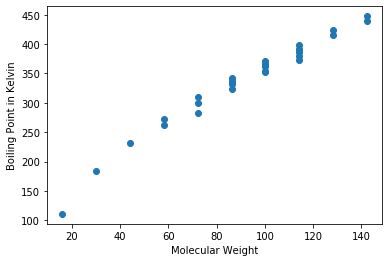Clearly from the data we can see that we have multiple molecules with the same molecular weight, but different boiling points. Molecular weight is therefore not the best predictor of boiling point. We can see if there are other descriptors that we can use such as Weiner or Zagreb. Let’s add various descriptors to the dataframe.

### Adding descriptors to the dataset

We can now calculate the Wiener and Zagreb indices for each of our hydrocarbons and add them to the dataframe.

In :

# create new lists to store results we calculate
result_Wiener= []
result_Z1= []
result_Z2= []

for index, row in df.iterrows():                # iterate through each row of the CSV data
SMILE = row['SMILES']                       # get SMILES string from row
mol = Chem.MolFromSmiles(SMILE)             # convert smiles string to mol file
result_Wiener.append(wiener_index(mol))     # calculate Wiener index descripter value
result_Z1.append(zagreb_index1(mol))        # calculate zagreb (Z1) descriptor value
result_Z2.append(zagreb_index2(mol))        # calculate zagreb (Z2) descriptor value

df['Wiener'] = result_Wiener           # add the results for WienerIndex to dataframe
df['Z1'] = result_Z1                   # add the results for Zagreb 1 to dataframe
df['Z2'] = result_Z2                   # add the results for Zagreb 2 to dataframe
df                                     # print the updated dataframe


Out:

compound name BP_C BP_K SMILES MW Wiener Z1 Z2
0 1 Methane -162.2 110.95 C 16.043 0 0.0 0.0
1 2 Ethane -88.6 184.55 CC 30.070 1 2.0 1.0
2 3 propane -42.2 230.95 CCC 44.100 4 6.0 4.0
3 4 butane -0.1 273.05 CCCC 58.120 10 10.0 8.0
4 5 2-methylpropane -11.2 261.95 CC(C)C 58.120 9 12.0 9.0
5 6 pentane 36.1 309.25 CCCCC 72.150 20 14.0 12.0
6 7 2-methylbutane 27.0 300.15 CC(C)CC 72.150 18 16.0 14.0
7 8 2,2-dimethylpropane 9.5 282.65 CC(C)(C)C 72.150 16 20.0 16.0
8 9 hexane 68.8 341.95 CCCCCC 86.180 35 18.0 16.0
9 10 2-methylpentane 60.9 334.05 CC(C)CCC 86.180 32 20.0 18.0
10 11 3-methylpentane 63.3 336.45 CC(CC)CC 86.180 31 20.0 19.0
11 12 2,2-dimethylbutane 49.8 322.95 CC(C)(CC)C 86.180 28 24.0 22.0
12 13 2,3-dimethylbutane 58.1 331.25 CC(C)C(C)C 86.180 29 22.0 21.0
13 14 heptane 98.5 371.65 CCCCCCC 100.200 56 22.0 20.0
14 15 3-ethylpentane 93.5 366.65 C(C)C(CC)CC 100.200 48 24.0 24.0
15 16 2,2-dimethylpentane 79.2 352.35 CC(C)(CCC)C 100.200 46 28.0 26.0
16 17 2,3-dimethylpentane 89.8 362.95 CC(C)C(CC)C 100.200 46 26.0 26.0
17 18 2,4-dimethylpentane 80.6 353.75 CC(C)CC(C)C 100.200 48 26.0 24.0
18 19 2-methylhexane 90.1 363.25 CC(C)CCCC 100.205 52 24.0 22.0
19 20 3-methylhexane 91.8 364.95 CC(CC)CCC 100.200 50 24.0 23.0
20 21 octane 125.6 398.75 CCCCCCCC 114.230 84 26.0 24.0
21 22 3-methylheptane 118.9 392.05 CC(CC)CCCC 114.232 76 28.0 27.0
22 23 2,2,3,3-tetramethylbutane 106.5 379.65 CC(C)(C(C)(C)C)C 114.230 58 38.0 40.0
23 24 2,3,3-trimethylpentane 114.7 387.85 CC(C)C(CC)(C)C 114.230 62 34.0 36.0
24 25 2,3,4-trimethylpentane 113.7 386.85 CC(C)C(C(C)C)C 114.230 65 32.0 33.0
25 26 2,2,4-trimethylpentane 99.3 372.45 CC(C)(CC(C)C)C 114.230 66 34.0 32.0
26 27 nonane 150.7 423.85 CCCCCCCCC 128.250 120 30.0 28.0
27 28 2-methyloctane 143.0 416.15 CC(C)CCCCCC 128.259 114 32.0 30.0
28 29 decane 174.2 447.35 CCCCCCCCCC 142.280 165 34.0 32.0
29 30 2-methylnonane 166.9 440.05 CC(C)CCCCCCC 142.280 158 36.0 34.0

Now we can see how each of these descriptors are related to the boiling points of their respective compounds.

In :

plt.scatter(df.Wiener, df.BP_K) # plot of BP versus Wiener index
plt.xlabel('Wiener Index')
plt.ylabel('Boiling Point in Kelvin')
plt.show()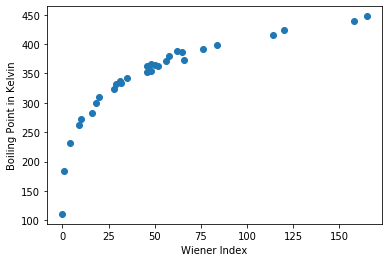In :

plt.scatter(df.Z1, df.BP_K) # plot of BP versus Zagreb M1
plt.xlabel('Zagreb M1')
plt.ylabel('Boiling Point in Kelvin')
plt.show()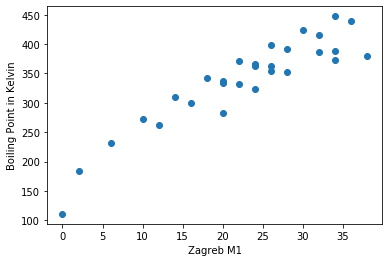In :

plt.scatter(df.Z2, df.BP_K) # plot of BP versus Zagreb M2
plt.xlabel('Zagreb M2')
plt.ylabel('Boiling Point in Kelvin')
plt.show()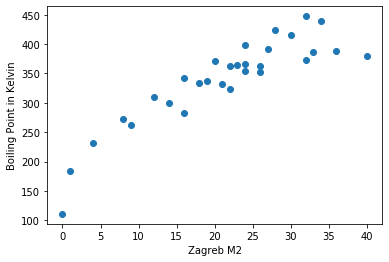Clearly molecular weight was somewhat predictive, but problematic. It looks like using the other indicators we have have some other ways to predict boiling point.

One option is write this data to a new CSV file and work in Microsoft Excel to perform a regression analysis. Exporting the data is straightforward and your instructor may provide instructions on how to analyze the data using Excel.

In :

df.to_csv('bp_descriptor_data.csv', encoding='utf-8', index=False)


## Mulitple regression analysis using statsmodels

The statsmodels package provides numerous tools for performaing statistical analysis using Python. In this case, we want to perform a multiple linear regression using all of our descriptors (molecular weight, Wiener index, Zagreb indices) to help predict our boiling point.

In :

import statsmodels.api as sm           # import the statsmodels library as sm
X = df[["MW", "Wiener", "Z1", "Z2"]]   # select our independent variables
y = df[["BP_K"]]                       # select BP as our dependent variable
model = sm.OLS(y,X).fit()              # set up our model
predictions = model.predict(X)         # make the predictions
print(model.summary())                 # print out statistical summary

C:\ProgramData\Miniconda3\envs\OLCC2019\lib\site-packages\numpy\core\fromnumeric.py:2389: FutureWarning: Method .ptp is deprecated and will be removed in a future version. Use numpy.ptp instead.
return ptp(axis=axis, out=out, **kwargs)

                            OLS Regression Results
==============================================================================
Dep. Variable:                   BP_K   R-squared:                       0.994
Method:                 Least Squares   F-statistic:                     1124.
Date:                Wed, 16 Oct 2019   Prob (F-statistic):           8.16e-28
Time:                        19:04:48   Log-Likelihood:                -93.019
No. Observations:                  30   AIC:                             196.0
Df Residuals:                      25   BIC:                             203.0
Df Model:                           4
Covariance Type:            nonrobust
==============================================================================
coef    std err          t      P>|t|      [0.025      0.975]
------------------------------------------------------------------------------
const         55.5695      6.745      8.238      0.000      41.677      69.462
MW             4.4325      0.203     21.853      0.000       4.015       4.850
Wiener        -0.6411      0.064     -9.960      0.000      -0.774      -0.509
Z1            -4.3920      1.238     -3.549      0.002      -6.941      -1.843
Z2             0.2982      0.943      0.316      0.754      -1.644       2.240
==============================================================================
Omnibus:                        3.756   Durbin-Watson:                   1.285
Prob(Omnibus):                  0.153   Jarque-Bera (JB):                2.259
Skew:                          -0.583   Prob(JB):                        0.323
Kurtosis:                       3.671   Cond. No.                         755.
==============================================================================

Warnings:
 Standard Errors assume that the covariance matrix of the errors is correctly specified.


Note above that we now have coeffiecients for an equation that can be used for prediction of boiling points for molecules not included in our dataset. The equation would be:

Predicted BP = 4.4325 * MW - 0.6411 * Weiner - 4.3920 * Z1 + 0.2982 * Z2 + 55.5695



We can use this equation to predict the boiling point of a new molecule. However, before we do, we need to explore the validity of the model.

### Model summary and analysis using partial regression plots

A quick look at the results summary shows that the model has an excellent R-squared value. Upon more careful examination, you may notice that one of our descriptors has a very large P value. This would indicate that perhaps the Z2 descriptor is not working well in this case. We can generate a more graphical interpretation that will make this more obvious.

In :

fig = plt.figure(figsize=(10,8))
fig = sm.graphics.plot_partregress_grid(model, fig=fig)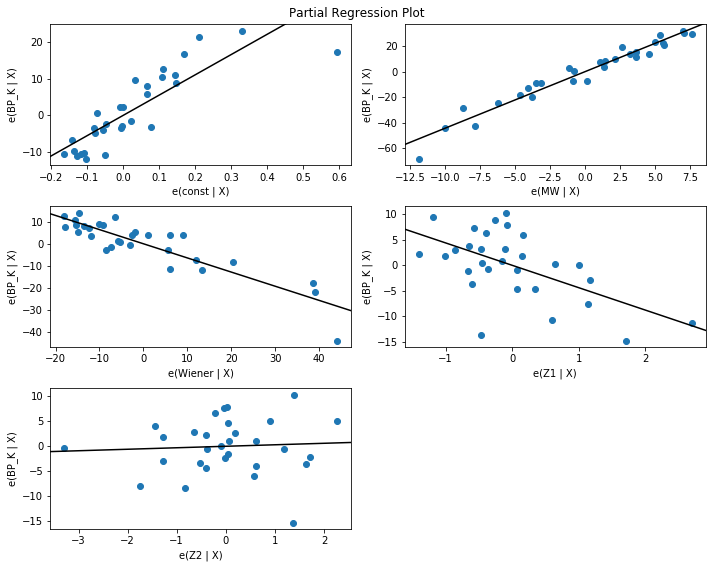Part of the reason that Z2 may not be predictive in this model is that there is colinearity with the Z1 descriptor. Both descriptors have similar calculations (as outlined in the Libretexts page for this activity). Later on in this exercise we can explore dropping this descriptor.

### How good is our model?

If we look at a plot of actual versus predicted boiling points

In :

pred_bp = model.fittedvalues.copy()       # use our model to create a set of predicted bp's
fig, ax = plt.subplots(figsize=(8, 5))
lmod = sm.OLS(pred_bp, df.BP_K)           # linear regression of observed vs predicted bp's
res = lmod.fit()                          # run fitting
plt.scatter(pred_bp, df.BP_K)             # plot of of observed vs predicted bp's
plt.ylabel('observed boiling point (K)')
plt.xlabel('predicted boiling point (K)')
plt.show()
print(res.summary())                      # print linear regression stats summary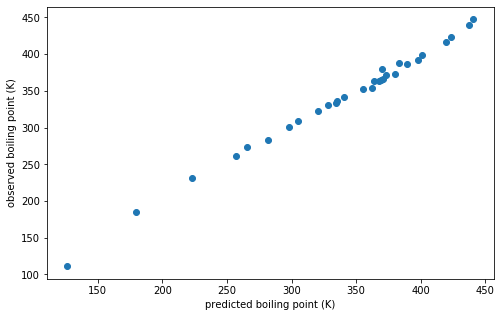OLS Regression Results
=======================================================================================
Dep. Variable:                      y   R-squared (uncentered):                   1.000
Model:                            OLS   Adj. R-squared (uncentered):              1.000
Method:                 Least Squares   F-statistic:                          1.213e+05
Date:                Wed, 16 Oct 2019   Prob (F-statistic):                    4.52e-54
Time:                        19:05:22   Log-Likelihood:                         -93.015
No. Observations:                  30   AIC:                                      188.0
Df Residuals:                      29   BIC:                                      189.4
Df Model:                           1
Covariance Type:            nonrobust
==============================================================================
coef    std err          t      P>|t|      [0.025      0.975]
------------------------------------------------------------------------------
BP_K           0.9998      0.003    348.263      0.000       0.994       1.006
==============================================================================
Omnibus:                        3.635   Durbin-Watson:                   1.284
Prob(Omnibus):                  0.162   Jarque-Bera (JB):                2.167
Skew:                           0.574   Prob(JB):                        0.338
Kurtosis:                       3.643   Cond. No.                         1.00
==============================================================================

Warnings:
 Standard Errors assume that the covariance matrix of the errors is correctly specified.


The model appears to have very good predictability (R-squared = 1.000) within the original 30 molecule data set. One way to test this model is to use a new molecule with its descriptors to see how well it is predicted. One molecule in the dataset is 2-methylheptane. It has the following data: MW = 114.232 Wiener Index = 79 Z1 = 28 Z2 = 26 Boiling Point = 390.6 K

Using the equation from above we can determine that the boiling point from the equation Predicted BP = 4.4325 MW - 0.6411 Weiner - 4.3920 Z1 + 0.2982 Z2 + 55.5695 is 396.0 K. The model gives a 1.4% error for prediction of the boiling point outide the training set.

We had mentioned earlier that Z2 may not be very predictive in this model. We can remove the variable an rerun the analysis to see if we can improve the predictability of the model.

In :

import statsmodels.api as sm           # import the statsmodels library as sm
X = df[["MW", "Wiener", "Z1"]]         # select our independent variables, this time without Z2
y = df[["BP_K"]]                       # select BP as our dependent variable
model = sm.OLS(y,X).fit()              # set up our model
predictions = model.predict(X)         # make the predictions
print(model.summary())                 # print out statistical summary

                            OLS Regression Results
==============================================================================
Dep. Variable:                   BP_K   R-squared:                       0.994
Method:                 Least Squares   F-statistic:                     1552.
Date:                Wed, 16 Oct 2019   Prob (F-statistic):           1.99e-29
Time:                        19:05:30   Log-Likelihood:                -93.078
No. Observations:                  30   AIC:                             194.2
Df Residuals:                      26   BIC:                             199.8
Df Model:                           3
Covariance Type:            nonrobust
==============================================================================
coef    std err          t      P>|t|      [0.025      0.975]
------------------------------------------------------------------------------
const         55.4979      6.624      8.378      0.000      41.882      69.113
MW             4.4114      0.188     23.433      0.000       4.024       4.798
Wiener        -0.6397      0.063    -10.139      0.000      -0.769      -0.510
Z1            -4.0260      0.430     -9.364      0.000      -4.910      -3.142
==============================================================================
Omnibus:                        2.917   Durbin-Watson:                   1.326
Prob(Omnibus):                  0.233   Jarque-Bera (JB):                1.624
Skew:                          -0.507   Prob(JB):                        0.444
Kurtosis:                       3.521   Cond. No.                         741.
==============================================================================

Warnings:
 Standard Errors assume that the covariance matrix of the errors is correctly specified.


IThe model appears to have very good predictability (R-squared = 0.994) within the original 30 molecule data set. Let's reexamine 2-methylheptane: MW = 114.232 Wiener Index = 79 Z1 = 28 Boiling Point = 390.6 K

Using the equation from above we can determine that the boiling point from the equation Predicted BP = 4.4114 MW - 0.6397 Weiner - 4.0260 * Z1 + 55.4979 is 396.2 K. The model also gives a 1.4% error for prediction of the boiling point outide the training set.

We can see here that Z2 doesn't really change the result of the calculation, and can probably be best left out to simplify the model.

Keep in mind that we have completed this analysis with only a training set of 30 molecules. If the training set had more molecules, you should be able to develop a better model.

## Assignment

You originally ran this analysis on a 30 molecule data set (BP.CSV). You also have available to you a 102 molecule data set (102BP.CSV).

• Complete the above analysis using the expanded data set to determine if a better predictive model can be obtained with a larger training set. Note that 2-methylheptane is in this new dataset so you will need to choose a new test molecule.

When you have completed the analysis, you will create a new analysis:

• Choose four new topological and other calculated descriptors found in Mordred http://mordred-descriptor.github.io/documentation/master/api/modules.html

• Complete simple linear analysis for each of your new descriptors.

• Complete a multiple linear regression to create an equation that best represents the data boiling point data and your descriptors.
• Create a separate sheet that has your regression data.
• Make a plot of Actual vs Predicted BP for your regression.
• Choose a new molecule not in the dataset (not 2-methylheptane, be creative and use chemical intuition).
• Use your multiple linear equation to predict this molecule’s BP and look of the literature value.
• Write a short one-two page paper that includes:
• What your new chosen descriptors mean
• Which new chosen descriptors correlate
• What is the overall equation calculated
• How to choose the molecule to test
• How close this multiple linear regression predicts your boiling point of your molecule

5.4: Python Assignment is shared under a not declared license and was authored, remixed, and/or curated by LibreTexts.